# Computational methods in chemistry

## Chemist Mark Tuckerman on the laws of motion, observables, and the Monte-Carlo approach

### videos | September 28, 2015

What do galaxies and atoms have in common? How can temperature be found given the data about microscopic motion? What is the first principles approach? These and other questions are answered by Professor of Chemistry and Mathematics at New York University Mark Tuckerman.

Computational methods in chemistry – this is really a subject that is interdisciplinary in the sense that it brings together physics and chemistry and mathematics and computer science, all under one umbrella, if you will. The topic is as old as the laws of physics are themselves. It goes back all the way to the formulation of the laws of classical mechanics by Sir Isaac Newton, so that brings us back to the 16th century. At that time, what people were interested in was predicting the specific motion of celestial bodies. So, they wanted to know how to find the trajectories of planets and stars and things under the action of the gravitational attractive forces that govern their motion. What was discovered in the mid-20th century, we think, about the late 50s, that those very same laws that govern the motions of planets and stars and even galaxies, joined celestial bodies, could also be applied to microscopic world with some strong caveats about these being approximate. But they could be applied there to tell us about the motions of atoms and molecules.Chemist Mark Tuckerman on classical nucleation theory, rare events phenomena, and energetic balance in the melting process
So, the very same laws that govern the motions of very large things could also then be applied to tell us about the motion of very small things. The difference is that, in the case of celestial bodies: planets and such, we know what the basic law of action is; we know what the forces are. These are going to be the forces of gravitational attraction. At the level of atoms and molecules the forces are rather more complicated, there is a variety of forces that act, they are all of an electrostatic nature, so what that means is that when you go to the microscopic world, the force laws are governed by rules that are attractive if two charges are unlike and they are repulsive if the charges are alike. So when we have charged nuclei and electrons, these are the kinds of forces that we have to deal with. These are often then turned into effective forces that give rise to weak type of attractions, what we call van der Waals’ interactions and stronger attractions that give rise to things like hydrogen bonding and then very strong attractions that actually lead to chemical bonding. And all of these forces must be described within our laws of motion.

So, what we do is we create effective models for these forces and then we use them in the laws of physics, in Newton’s laws, to tell us how the atoms in the molecules move around and, therefore, that will give us the motions of the molecules themselves. When we do that, of course, we can’t get exact solutions to the equations of motion, because they are very-very complicated, but we can do this using computational techniques. So computers actually allow us to generate solutions to these laws of motion. What we do then is we take the laws of motion and we simply come up with an algorithm for discretizing them.

So, in time, which is what the laws of motion are giving us, they are giving us the motions of atoms and molecules in time, what we do then is we discretize these equations in time and then we allow a computer to generate approximate solutions to the equations of motion.

And they will tell us about all sorts of processes that happen in the microscopic world, they will tell us about, in principle, chemical bond breaking, so we can make chemical reactions, they will tell us about how complex systems such as proteins and nucleic acids, which are important in biology, how they fold into their biologically relevant conformations, they will tell us about complex membranes, for example, of industrial importance, things that go into batteries and fuel cells, how they will fold into their chemically active conformations, they will tell us about things like how small molecules move around, so how small molecules might diffuse in biological systems and a whole variety of interesting processes like this. And all of this is encoded in these laws of motion.

Usually, the systems that we want to study are sufficiently complex that we need very large computers in order to do our calculations. So, many of the computers that we use are high-performance computing platforms, they have many-many processors operating in parallel, and we let them simply operate on the laws of motion, and then we can handle systems consisting of, typically, maybe millions or even tens of millions of atoms, which is large enough that we can start to approximate real systems, but, of course, small, on the scale of what you might consider actual macroscopic matter. Nevertheless, what we get from this type of calculation is still relevant and can give us properties that can actually be measured in experiments, which is really what we want to do, we want to be able to predict the results of experiments that may yet be performed or we want to be able to rationalize the results of experiments that have been performed and try to understand them from the point of view of this microscopic-detailed motion.

So, there are important approximations that we’re making when we assume that the basic classical laws of motion can be applied at the microscopic level. The most important one, and this is an important one, is that really, at the level of atoms and molecules, the laws of quantum physics really govern what’s going on, and when we use the laws of Newton, the classical laws of motion, well, we’re neglecting the quantum effects that are there. So that is a severe approximation, it is the one that can be corrected for with techniques that are not unlike what one uses when applying the classical laws of physics, but they effectively incorporate quantum effects that we’re missing. So that’s something that we always have to be aware of that we’re making this approximation that the laws of classical physics apply rather than the laws of quantum physics. But once we accept that approximation, then we can turn all this microscopic data that we get, which is really at the level of having the time-dependent trajectories of the exact motion of every atom that we’re studying in our system. And we need to turn that into something that can actually be measured in an experiment, so we need to turn that into what we call an observable.

The way we do that is to use rules of something called ‘statistical mechanics’, statistical mechanics is an area that is applied throughout physics and chemistry and even biology to connect all this microscopic detail that we have, all these time-dependent trajectories of thousands, hundreds of thousands, even millions of atoms in our system into real observables. What it says, basically, is that you can attach to all this motion a specific statistical distribution, typically, a very simple one, and then you can express any observable that you might be interested in as an average over such a distribution. And using those rules, you can turn all this microscopic motion into an actual physical quantity that we might want to measure. A very simple example would be to turn the, let’s say, all the velocities of all the atoms into something that you might measure, which would be the temperature of the system. That could be done simply by computing an average of the squares of all those velocities times their masses, which is a measure of the kinetic energy, and that connects directly to temperature. So, temperature, which is something you can get just by putting a thermometer in your system, can be obtained simply by doing this kind of average over the velocities.

So, other things that we can obtain from all this microscopic motion might include, for example, the pressure of the system, which is something that you can easily measure, you might want to get things like entropy or the enthalpy or just average energies.

We might be interested in dynamical properties, that would include rates of diffusion, rates of chemical reactions, various vibrational properties, all of these things are available to us through the application of the laws of statistical mechanics to all of this underlying motion that we get from our computer simulations.

I should mention of course that molecular dynamics, which is this technique by which we’re solving the Newtonian equations numerically, there’s an alternate way to get statistical properties and observable properties, and it’s through a method known as Monte-Carlo, which is another very popular approach.

Again, like molecular dynamics, Monte-Carlo was an approach that originated, maybe, around the mid-20th century or so, and the idea here is that instead of using the statistical distribution to turn the microscopic trajectories into observables, we actually work directly with that distribution and we create samples from it. So the way that’s done, and hence the name Monte-Carlo, is that it’s done through what we call “Games of chance”. Well, the idea here is to use random numbers in a particular model “game of chance” to create samples, direct samples from this statistical distribution that you’re interested in. You create these samples, and what these samples then are are microscopic configurations, so, in other words, they would be configurations that are the positions of every atom in your system, for example, or every molecule in your system. You choose a level of course training that you want, and then the rules of Monte-Carlo give you these configurations. Then, you can perform direct averages over these configurations in much the same way that I’ve just described and turn those also into the observable properties of the system.

Where the field is now is, of course, we have very good models for the interactions between atoms and molecules in the system, so we can model these things reasonably accurately. But there’s a better way to do it than using effective models. These models tend to describe, in very simple ways, chemical bonding and they tend to describe very localized motions of atoms in molecules, for example, if I have 3 atoms connected by 2 chemical bonds, then there might be a bending motion between them, and if I have 4 atoms connected by chemical bonds, then there’s going to be a sort of torsional motion between them.

All these various things can be described in very-very simple models for these types of interactions, but a much better way to treat this kind of problem, to obtain these interactions is to use the rules of quantum mechanics. I said that we approximate the system with the laws of classical physics, but where the forces come from if we don’t want to use a model is we can use a level of quantum mechanics that separates the electrons from the nuclei and says well, we know that electrons are, basically, the glue that holds atoms together into molecules, it’s electrons that are responsible for chemical bonding, it’s electrons that are responsible for weak interactions such as van der Waals forces and things like that. So, the alternate way then is to treat the electron distribution and the quantum electronic structure problem directly, solve that problem and then from the solution of that problem obtain the forces between nuclei directly from this electronic structure. When you do that, you’re doing something that’s called first principles, or, using the Latin phrase, ab initio, molecular dynamics, which means from the first principles.

What this kind of model can describe that many of these other effective models can’t, is actual chemical bond breaking and forming events, you can really do chemistry this way. And, of course, the other thing that you get from this is the change, the changing distribution, so you’ve got a way of describing the interactions that are sensitive to the local environment and how that local environment changes as atoms and molecules change their configurations as they move around, and this is actually important for having a higher degree of accuracy in the model and also to be able to predict things without having to have the benefit of a model that may have certain parameters that you need to adjust according to the experiments that you’re trying to mimic. So, this actually gives you a way to model the system and with it’s independent of the experiments and then to predict something that you might not have experimental information about, in other words, to be able to predict the results of an experiment that is yet to be done.

So, with effective models, I may have mentioned that you can do systems on the order of thousands, tens of thousands, even millions of atoms, but if you use this first principles approach, then you’re, sort of, limited to systems on the order of maybe a hundred atoms or so, which is considerably smaller. At the same time, the kinds of timescales that you can reach with these effective models may be on the order of microseconds or milliseconds if you have a very good computer, or, depending on the complexity of the system, may be nanoseconds, or 10 to the -9 seconds. If you use this first principles approach, you’re limited typically to, maybe, about 10 to the -12, what’s called a picosecond, up to, perhaps, 10 or even a hundred picoseconds at most.

So, where the field wants to go, of course, from here is to be able to have algorithms that are, effectively, able to attain these interactions from first principles more efficiently and, of course, take advantage of emerging hardwares: graphical processing units and very large-scale parallel computers to be able to extend both the time and the length scales so that we have the ability to predict these results on larger systems more accurately for longer times. The longer the time we can get, the more interesting processes we’re able to mimic.Professor of Chemistry and Mathematics, New York University, NYU-ECNU Center for Computational Chemistry at NYU Shanghai
Did you like it? Share it with your friends!
Published items
0779
To be published soon
+92

#### Most viewed

• 1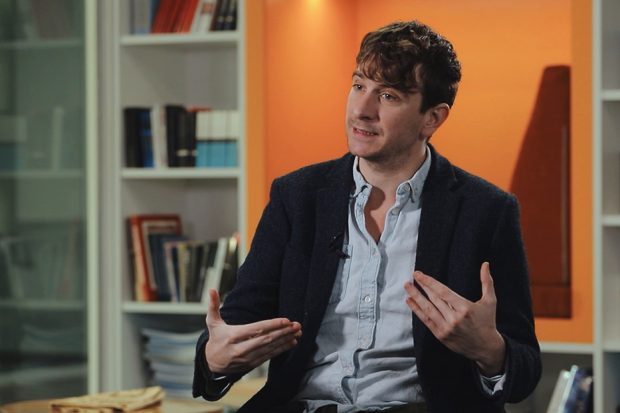Peter Jones
• 2John Worrall
• 3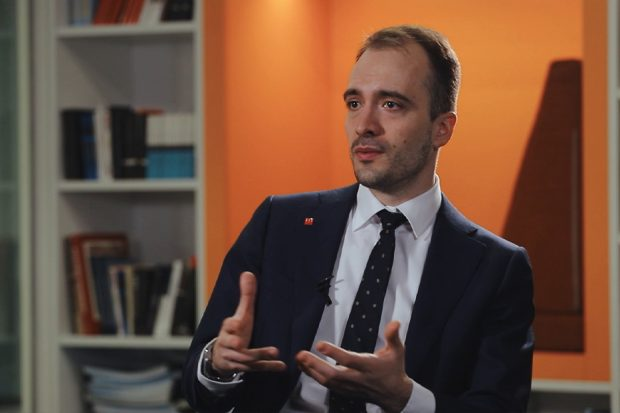Daniel Kontowski
• 4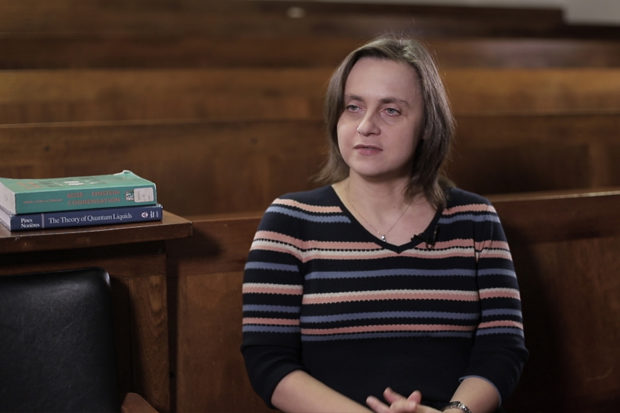Marzena Szymanska
• 5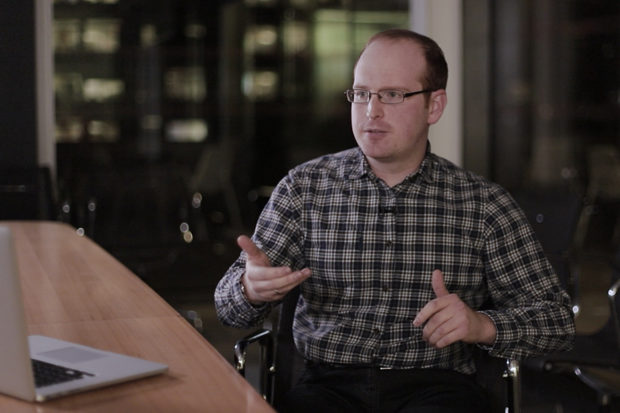Jonathan Birch
• 6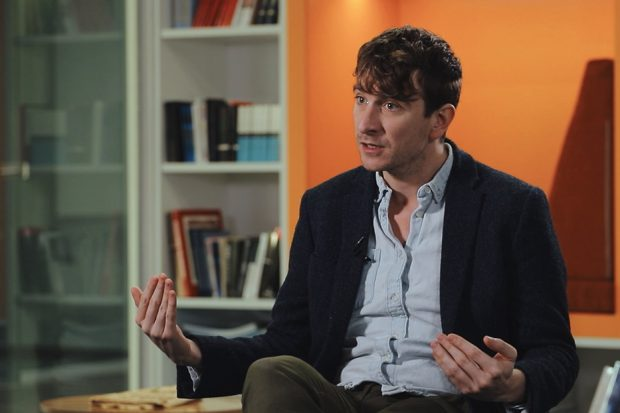Peter Jones
• 7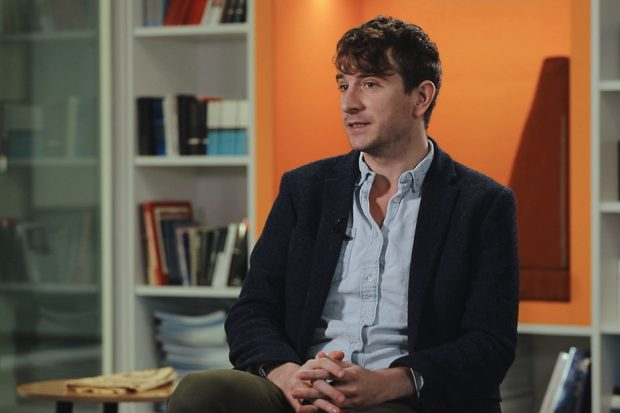Peter Jones
• 8Jeffrey Taylor
• 9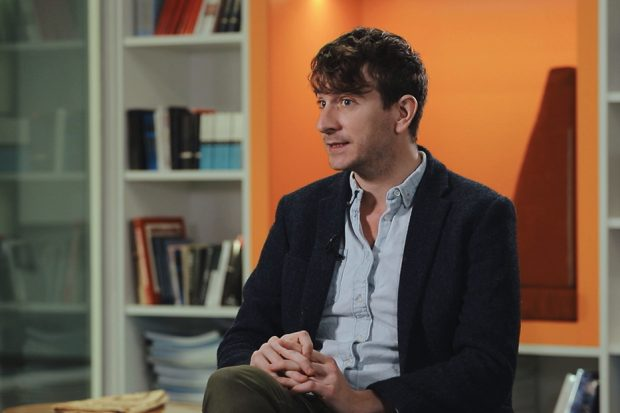Peter Jones
•